Home > ACC7 > Chapter cc38 > Lesson cc38.2.3 > Problem8-95

8-95.
1. Simplify each expression using the rules for exponents. Homework Help ✎

1.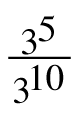2. 10x4(10x)−2

3.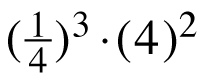4.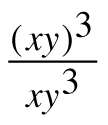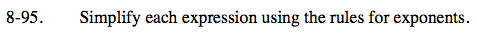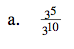3(5 − 10)

$3^{-5}=\frac{1}{3^{5}}$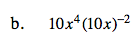Remember, when an exponent is located outside of a group of parentheses, the exponent applies to each and every value within the parentheses.

10x4(10−2x−2)

$\frac{{\it x}^{2}}{10}$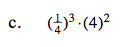This can be rewritten as:

(4−1)3(4)2

See (b).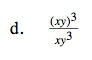See (a) and (b).